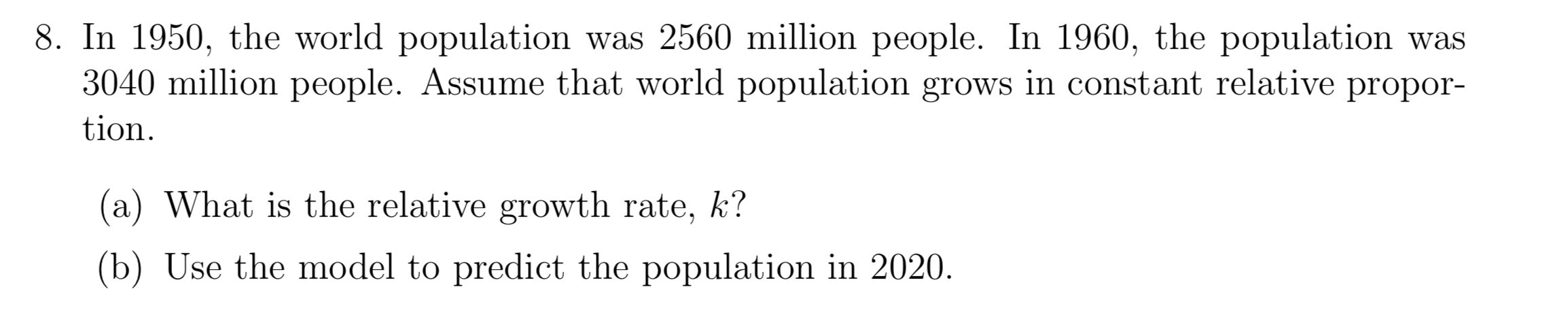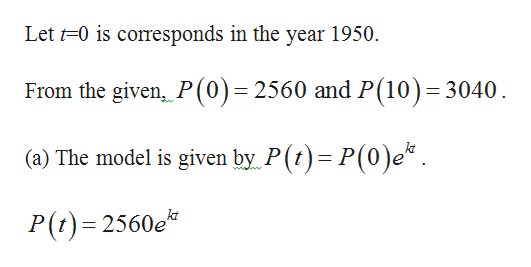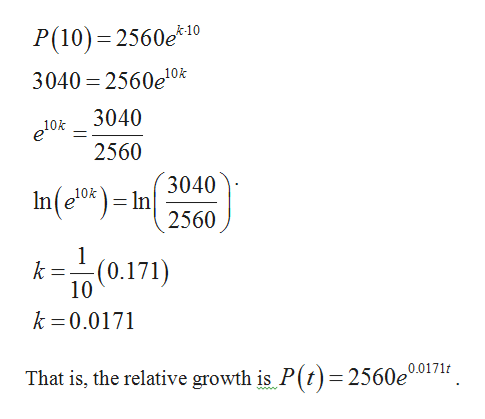# 8. In 1950, the world population was 2560 million people. In 1960, the population was3040 million people. Assume that world population grows in constant relative propor-tion(a) What is the relative growth rate, k?(b) Use the model to predict the population in 2020.

Questionhelp_outlineImage Transcriptionclose8. In 1950, the world population was 2560 million people. In 1960, the population was 3040 million people. Assume that world population grows in constant relative propor- tion (a) What is the relative growth rate, k? (b) Use the model to predict the population in 2020. fullscreen
check_circle

Step 1

Consider P(t) is the population in the year t.help_outlineImage TranscriptioncloseLet 0 is corresponds in the year 1950 From the given, P(0)=2560 and P(10) 3040 (a) The model is given by P(t) P(0)e* P(t) 2560e fullscreen
Step 2

At t=10,...help_outlineImage TranscriptioncloseP(10) 2560e*10 3040 2560e10k 3040 e10k 2560 3040 In(el0) In 10k 2560 (0.171) k 10 k 0.0171 0.0171t That is, the relative growth is P(t)= 2560e' fullscreen

### Want to see the full answer?

See Solution

#### Want to see this answer and more?

Solutions are written by subject experts who are available 24/7. Questions are typically answered within 1 hour.*

See Solution
*Response times may vary by subject and question.
Tagged in

### Other